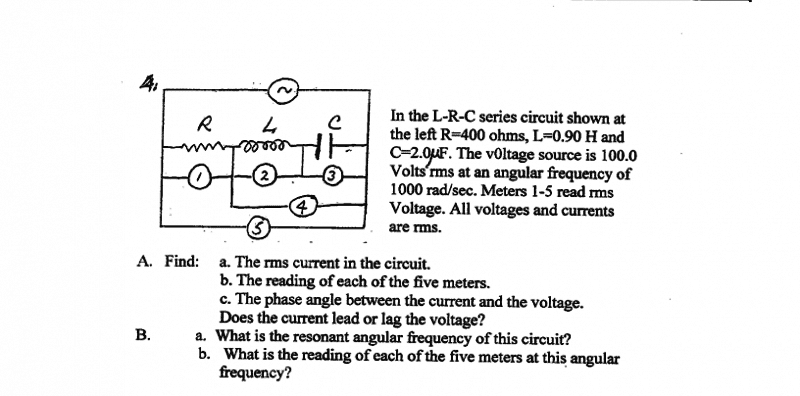LRC circuitHomework Equations

XL = ωL
XC = 1/ωC
Z= sqrt(R^2+(XL-XC)^2)
∅ = tan^-1(XL-XC/R)

The Attempt at a Solution

A) a)
Irms = Vrms/R = 100 V/400 Ω = .25 A
b) 1) V= Vrms =100 V
2) V = IrmsXL = IrmsωL = (.25)(1000)(.9) = 225 V
3) V= IrmsXC = Irms/ωC = (.25)/((1000)(2E-6)) = 125 V
4) this part I am not sure how to do.
5) V = IrmsZ = Irmssqrt(R^2+(XL-XC)^2) = (.25)sqrt(400^2 + (900 - 500)^2) = 141 V

c) ∅=tan^-1(XL-XC/R) = tan^-1(400/400) = 45°
it is positive so voltage leads

B) a) ω = 1/sqrt(LC) = 1/sqrt(.9(2E-6)) = 745 rad/sec
b) 1) still 100 V
2) V = IrmsXL = IrmsωL = (.25)(745)(.9) = 168 V
3) V = IrmsXC = Irms/ωC = (.25)/((745)(2E-6)) = 168 V
4) ???
5) V = IrmsZ = IrmsR = .25(400) = 100 V

I am not entirely confident I did all of these right. feedback would be greatly appreciated

donpacino
Gold Member
your part a is wrong. I=V/Z, with Z being the impedance of the circuit. Since it is an AC waveform, the inductor and capacitor will have some impedance

so part a) would be I = V/Z = V/ sqrt(R^2 + (XL-XC)^2) = 100/sqrt(400^2 +(1000(.9-2E-6))^2) = .1 A ???

also would I be able to do part 4 using the formula V = IZ where the R in the formula for Z is set to 0?

donpacino
Gold Member
so part a) would be I = V/Z = V/ sqrt(R^2 + (XL-XC)^2) = 100/sqrt(400^2 +(1000(.9-2E-6))^2) = .1 A ???

also would I be able to do part 4 using the formula V = IZ where the R in the formula for Z is set to 0?
No.

What have you learned about AC circuits and inductors and capacitors?
Have you learned about the laplace transform yet?

I haven't heard of the laplace transform. Both of the things I said are wrong? I am still wrong about part a) ?

donpacino
Gold Member
the resistance at any given frequency for these purposes can be seen below
inducotor: w*L
capacitor: 1/(w*L)

now the inductor, capacitor,and resistor.... are they in series or parallel?

donpacino
Gold Member
the resistance at any given frequency for these purposes can be seen below
inducotor: j*w*L
capacitor: 1/(j*w*L)

now the inductor, capacitor,and resistor.... are they in series or parallel?

they are in series

donpacino
Gold Member
yup, so to find the total impedance, you add them together

I = V/Z = V/ sqrt(R^2 + (XL+XC)^2)
???
so when they are in parallel it is
1/XL + 1/XC ??

My book says XL-XC where does this come from?

donpacino
Gold Member
I = V/Z = V/ sqrt(R^2 + (XL+XC)^2)
???
so when they are in parallel it is
1/XL + 1/XC ??

My book says XL-XC where does this come from?
somehow I missed your equations page. oops

I forgot you havent really learned that much about AC so they gave you the equations.

http://en.wikipedia.org/wiki/Complex_plane

There are two ways to express complex numbers, polar and rectangular notation.
sqrt(R^2 + (XL+XC)^2) essentially converts the rectangular notation to the magnitude of polar notation
and ∅ = tan^-1(XL-XC/R) converts it to the angle of polar notation

donpacino
Gold Member
in that case, the second answer you gave is correct

the .1 A is correct for part a) ?

For part 4) is this a case where the Voltage oscillates?

donpacino
Gold Member
the .1 A is correct for part a) ?
yes
For part 4) is this a case where the Voltage oscillates?
do you mean finding the phase angle??
if yes then look at your equation for theta

I mean to find the voltage across the LC part of the circuit (If I am understanding the question correctly)
Originally I was thinking of using the equation for Z with R = 0 or
Z = sqrt((XL-XC)^2)
and then using
V = IZ

I am still trying to figure this out. Is this one of the cases where I have to use the formula for oscillating voltage? V=v0coswt ?

donpacino
Gold Member
recall each part has an impedance. you know what the impedance is

V=I*Z